• 3轴机械臂——MK2.zip 3D打印资料，开源资料，纯3D打印文件，
• 上一篇:grbl控制3轴机械臂 原理 实现 （一） 之2D机械臂模拟及实现 3轴机械臂几何分析 到目前为止，我们知道了如何在XY平面约束的情况下解决正逆运动学问题。从2D到3D的诀窍是重新使用2D解决方案。我们所介绍的...
参考文章:https://www.alanzucconi.com/2020/09/14/inverse-kinematics-in-3d/

上一篇:grbl控制3轴机械臂 原理 实现 （一） 之2D机械臂模拟及实现

3轴机械臂几何分析

到目前为止，我们知道了如何在XY平面约束的情况下解决正逆运动学问题。从2D到3D的诀窍是重新使用2D解决方案。我们所介绍的这种3轴机械臂，一共3个轴，控制L1臂一个轴，控制L2臂一个轴，底座将L1和L2臂整体旋转为一个轴。很明显我们就多了一个整体旋转的维度。并且底座的旋转不会改变L1和L2臂的角度。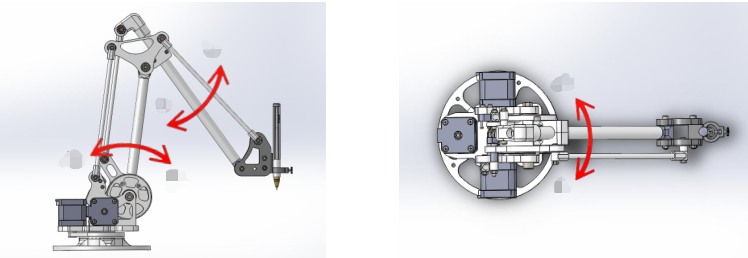我们可以通过三个步骤解决问题：

绕Y轴旋转目标点，直到它位于XY平面上
移动机械臂L1和L2臂，使其到达目标该点，就像在2D模式下一样
通过沿Y轴的相反方向旋转整个机械臂来“撤消”旋转。
以上三个步骤逆解举例：

假设我们最终要得到的目标点是下图红色点，那么我们就要求得大臂旋转角度，小臂旋转角度，两臂在Y轴旋转角度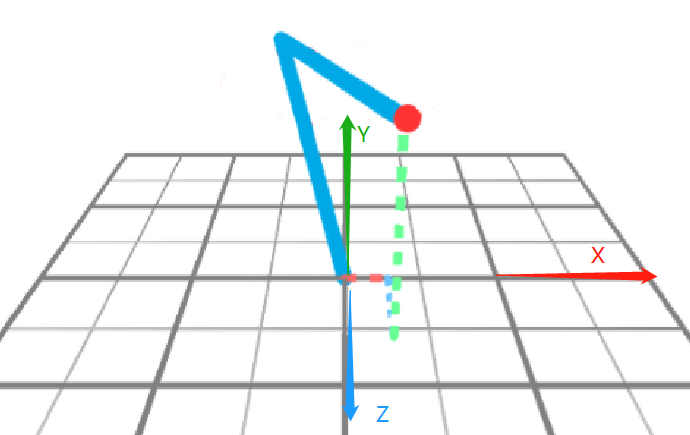根据步骤一，绕Y轴旋转到X，Y平面上如下图，这样我们就能像处理2D机械臂一样

步骤二，在此平面上处理得到的L1，L2臂角度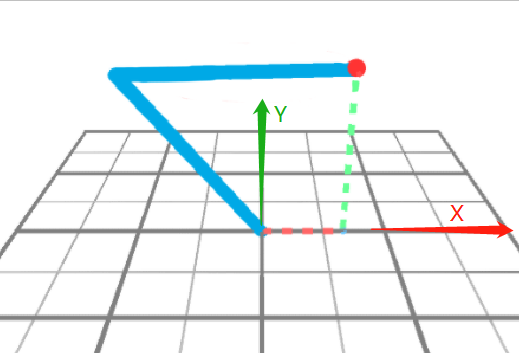步骤三，得到L1，L2臂的角度后，恢复Y轴的旋转，并求得Y轴的旋转角度

根据的值，求得紫色的线与X轴的夹角，这个夹角就是Y轴的旋转角度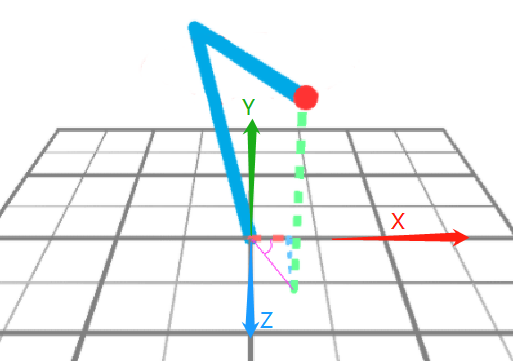至此，3个角度都求出来了。

实际上，旋转Y轴是为了方便理解，实际应用中，我们可以认为目标点所处于平面(W,Y)上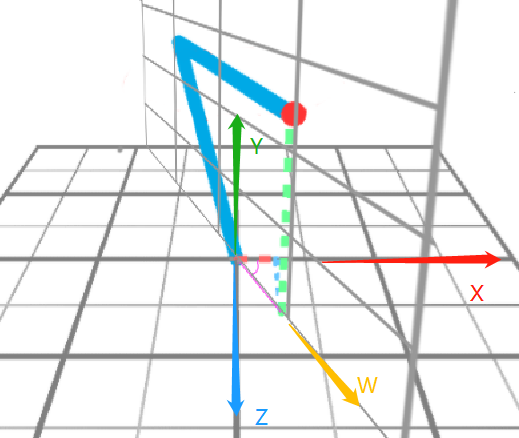在这个(W,Y)平面上，直接按2D机械臂方式来算出L1，L2臂的角度即可，Y轴旋转角度计算照旧。

3D运动学逆解（Inverse kinematics）

我们把2d机械臂的图画到3d里面，可见原来我们的X边，变成了W边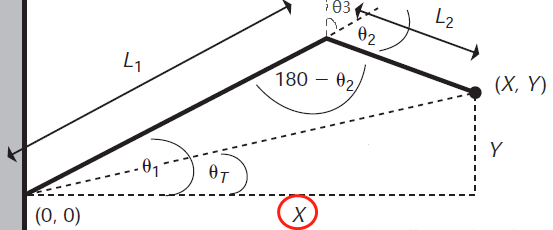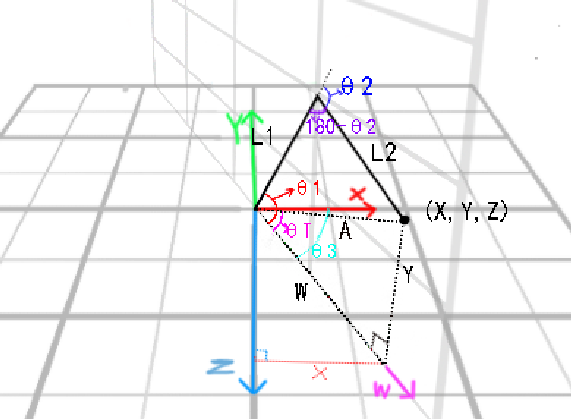根据坐标轴得知W边在（X，Z）平面，根据X边，W边，Z边是直角三角形，通过公式     求出W的长度

代入值得

得

再根据W边和Y边求出原点到（X,Y,Z）的距离为A边，W边，Y边，A边是直角三角形，依旧通过公式

代入值得

得        代入上面W得

接下来就和2d机械臂一样了

代入以上公式得

得

余弦定理公式：

根据余弦定理，将值代入公式得

同上根据余弦定理：

有：

是X轴到W边的角度，我们用三角函数来求

Z边，X边，W边组成一个直角三角形，则

得

这就求出3D机械臂的三个旋转角度了。

3D运动学正解（Forward kinematics）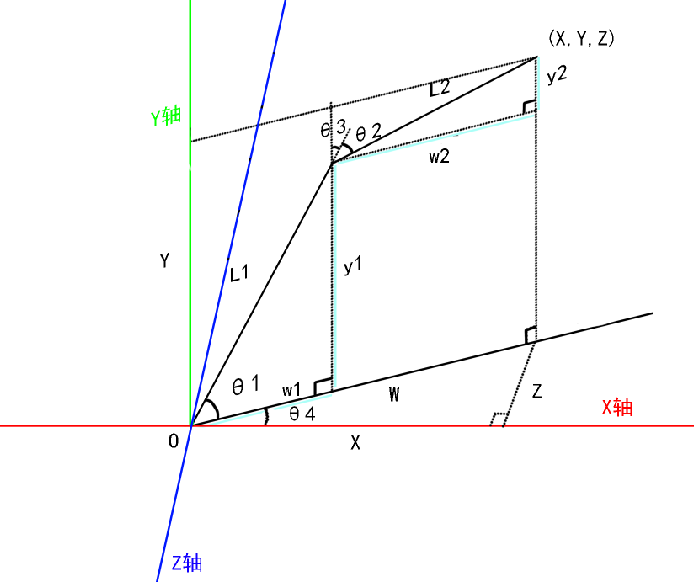对于3D机械臂的运动学正解，依旧可以和2D机械臂的方法。

首先我们已知L1，L2臂的臂长，L1，L2臂的角度和机械臂在Y轴旋转的角度，求坐标

首先和2D机械臂一样，我们先求X，Y的坐标，在这里就是W，Y平面，就是求W和Y的值。

w1,w2,y1,y2分别是浅蓝色线段部分

由于w1和w2是平行的，所以L1和w1所成角度，和L1和w2所成角度相同，所以有

根据三角函数:

则

代入上式得

代入上式得

然后根据W的值，求X和Z的值

得

得

至此求得X，Y，Z的值

软件模拟实现

这里用的unity进行的模拟，通过unity里面的父子组件关系，L1旋转可以带动其子组件L2一起旋转。所以一个臂是由两部分组成的joint和arm如图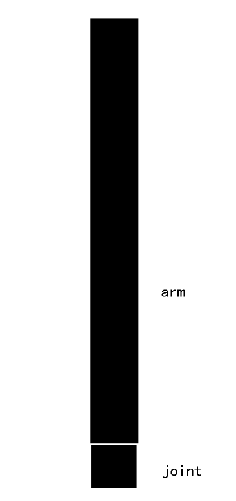这样把两个臂组装起来，再把他们绑在一个原点方块this上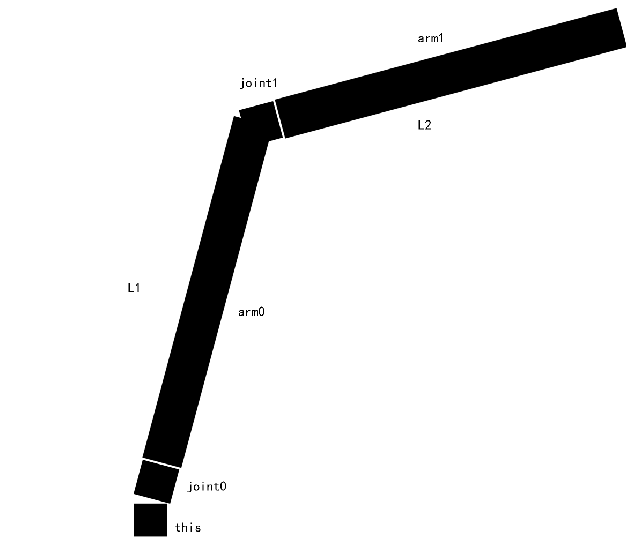它们的父子关系是

this
joint0
arm0
joint1
arm1

组合好后的样子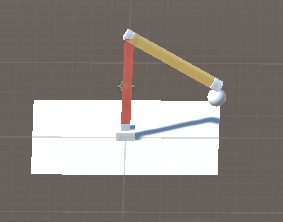此脚本放在this方块上，并把joint0，joint1,的transform拖到脚本的公共变量上

代码实现：

using System.Collections;
using System.Collections.Generic;
using UnityEngine;

public class ik : MonoBehaviour {

//[Header("Joints")]
public Transform Joint0;
public Transform Joint1;
public Transform Hand;

//[Header("Target")]
public Transform Target;

private float length0, L1;
private float length1, L2;

private float L1_2, L2_2;

private int X_AXIS = 0;
private int Y_AXIS = 1;
private int Z_AXIS = 2;

private float SCARA_OFFSET_X = 0;
private float SCARA_OFFSET_Y = 0.72f;
private float SCARA_OFFSET_Z = 0;

public bool angle_mode = false;

private float[] cartesian = new float, f_scara = new float;

void Start()
{
length0 = Vector3.Distance(Joint0.position, Joint1.position);
length1 = Vector3.Distance(Joint1.position, Hand.position);
L1 = length0;
L2 = length1;
L1_2 = sq(L1);
L2_2 = sq(L2);

}

// Update is called once per frame
void Update() {

float length2 = Vector3.Distance(Joint0.position, Target.position);

if (length2 > length0 + length1 - 0.3f)
{

}
else
{
cartesian = Target.position.x;
cartesian = Target.position.y;
cartesian = Target.position.z;

inverse_kinematics(cartesian, f_scara);

float[] cart = new float;
float[] scar = new float;

scar = (float [])f_scara.Clone();

forward_kinematics_SCARA(scar, cart);
Debug.Log("x:" + cart);
Debug.Log("y:" + cart);
Debug.Log("z:" + cart);
}

Joint0.transform.localEulerAngles = new Vector3(0, 0, 0);
Vector3 Euler0 = Joint0.transform.localEulerAngles;
Euler0.x = 90f - f_scara;
Joint0.transform.localEulerAngles = Euler0;

Joint1.transform.localEulerAngles = new Vector3(0, 0, 0);
Vector3 Euler1 = Joint1.transform.localEulerAngles;
Euler1.x = f_scara;
Joint1.transform.localEulerAngles = Euler1;

this.transform.localEulerAngles = new Vector3(0, 0, 0);
Vector3 Euler2 = this.transform.localEulerAngles;
Euler2.y = f_scara;
this.transform.localEulerAngles = Euler2;

}

float sq(float a)
{
return a * a;

}

// f_scara是大臂和小臂的角度，cartesian是坐标
void inverse_kinematics(float[] cartesian, float[] f_scara)
{
/***********************robot arm****************************/

//         y +  /z
//           | /
//           |/
//           +-----+x

float ROBOTARM_alpha, ROBOTARM_beta, ROBOTARM_cta, ROBOTARM_alphapsi, projectxyLength, X, Y, X_2, Y_2, sqrtx_2ay_2;

//首先求得目标点 到 原点的距离
//获取机械臂投射到xy平面的长度
//length = sqrt(x*x + y*y)
projectxyLength = Mathf.Sqrt(sq(cartesian[X_AXIS]) + sq(cartesian[Z_AXIS]));//对调yz,坐标系不同
//将3d机械臂变量变为2d机械臂的变量
//	projectxyLength长度为2d机械臂的X
X = projectxyLength;
X_2 = sq(X);
Y = cartesian[Y_AXIS];//对调yz,坐标系不同
Y_2 = sq(Y);
sqrtx_2ay_2 = Mathf.Sqrt(X_2 + Y_2);
//求得机械臂所在yz旋转平面的alphapsi角度
ROBOTARM_alphapsi = Mathf.Acos(X / sqrtx_2ay_2);
//如果坐标在平面以下，将alphapsi取反
if (Y < 0)
{
ROBOTARM_alphapsi = -ROBOTARM_alphapsi;
}

//求得机械臂所在yz旋转平面的alpha角度,，即大臂到xy平面的角度(实际是弧度)
ROBOTARM_alpha = Mathf.Acos((L1_2 + X_2 + Y_2 - L2_2) / (2 * L1 * sqrtx_2ay_2)) + ROBOTARM_alphapsi;
//求得小臂的角度(实际是弧度)
ROBOTARM_beta = Mathf.Acos((X_2 + Y_2 - L1_2 - L2_2) / (2 * L1 * L2));
//求得整体机械臂的旋转角度(实际是弧度)
ROBOTARM_cta = Mathf.Atan2(cartesian[X_AXIS], cartesian[Z_AXIS]);

//如果不是角度模式
if (!angle_mode)
{
f_scara[X_AXIS] = Mathf.Rad2Deg * ROBOTARM_alpha; //大臂旋转弧度转换为角度
f_scara[Y_AXIS] = Mathf.Rad2Deg * ROBOTARM_beta;   //小臂旋转弧度转换为角度
f_scara[Z_AXIS] = Mathf.Rad2Deg * ROBOTARM_cta;
}
//否则是角度模式
else
{
f_scara[X_AXIS] = cartesian[X_AXIS];
f_scara[Y_AXIS] = cartesian[Y_AXIS];
f_scara[Z_AXIS] = cartesian[Z_AXIS];

}
}

//传入值 f_scara是大臂和小臂的角度，cartesian是最终求得的坐标
void forward_kinematics_SCARA(float [] f_scara, float [] cartesian)
{
/***********************robot arm****************************/
float X, Y, Z;
float project3D;
//f_scara里面的0,1,2代表：大臂角度，小臂角度，旋转角度

//         y +  /z
//           | /
//           |/
//           +-----+x

//unity 角度空间转换转换
//f_scara[X_AXIS] = 90f - f_scara[X_AXIS];

//2D机械臂朝向的方向,两臂投影到平台平面长度
//Z = Mathf.Cos(Mathf.Deg2Rad * f_scara[X_AXIS]) * L1 + Mathf.Sin((Mathf.Deg2Rad * f_scara[Y_AXIS]) + (Mathf.Deg2Rad * 90) - (Mathf.Deg2Rad * f_scara[X_AXIS])) * L2;

//3D 两臂投影到平台平面长度,通过三角函数转化为坐标轴方向长度
project3D =  Mathf.Cos(Mathf.Deg2Rad * f_scara[X_AXIS]) * L1 + Mathf.Sin((Mathf.Deg2Rad * f_scara[Y_AXIS]) + (Mathf.Deg2Rad * 90) - (Mathf.Deg2Rad * f_scara[X_AXIS])) * L2;
Z = Mathf.Cos((Mathf.Deg2Rad * f_scara[Z_AXIS])) * project3D;

//垂直向上的方向
Y = Mathf.Sin((Mathf.Deg2Rad * f_scara[X_AXIS])) * L1 + Mathf.Cos((Mathf.Deg2Rad * f_scara[Y_AXIS]) + (Mathf.Deg2Rad * 90) - (Mathf.Deg2Rad * f_scara[X_AXIS])) * L2;

//2D 站机械臂后方看向它，它的右边方向
//X = Mathf.Sin((Mathf.Deg2Rad * f_scara[Z_AXIS])) * Mathf.Cos((Mathf.Deg2Rad * f_scara[X_AXIS])) * L1 + Mathf.Sin((Mathf.Deg2Rad * f_scara[Y_AXIS]) + (Mathf.Deg2Rad * 90) - (Mathf.Deg2Rad * f_scara[X_AXIS])) * L2;

//3D 站机械臂后方看向它，它的右边方向
X = Mathf.Sin((Mathf.Deg2Rad * f_scara[Z_AXIS])) * project3D;

cartesian[X_AXIS] = X + SCARA_OFFSET_X;  //求得机械手顶点的坐标
cartesian[Y_AXIS] = Y + SCARA_OFFSET_Y;  //求得机械手顶点的坐标
cartesian[Z_AXIS] = Z + SCARA_OFFSET_Z;

/***********************robot arm****************************/
}

}

实现效果演示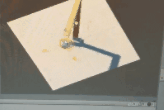接下来介绍下GRBL，以及如何在GRBL上控制机械臂，以及机械臂控制的硬件细节

下一篇:grbl控制3轴机械臂 原理 实现 （三） 之如何通过步进电机控制机械臂、插补算法


展开全文GRBL
• 机械臂简介 机械臂是指高精度，多输入多...因而机械臂的建模模型也存在着不确定性，对于不同的任务, 需要规划机械臂关节空间的运动轨迹，从而级联构成末端位姿 这里我们主要讲解臂杆型机械臂，如图 运动...
参考文章：https://blog.csdn.net/noahzuo/article/details/53908141

机械臂简介

机械臂是指高精度，多输入多输出、高度非线性、强耦合的复杂系统。因其独特的操作灵活性, 已在工业装配, 安全防爆等领域得到广泛应用。机械臂是一个复杂系统, 存在着参数摄动、外界干扰及未建模动态等不确定性。因而机械臂的建模模型也存在着不确定性，对于不同的任务, 需要规划机械臂关节空间的运动轨迹，从而级联构成末端位姿

这里我们主要讲解臂杆型机械臂，如图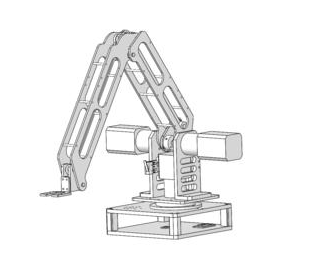运动原理

这里我们先从最简单的讲起，二维的机械臂如下图，这里分为L1大臂和L2小臂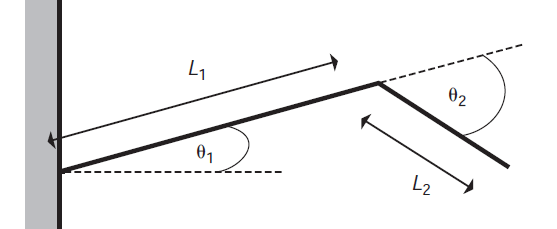那么这二维的机械臂的末端位置由大臂的角度和小臂的角度，以及这两臂的长所决定。

我们先假设大臂靠墙的坐标是（0,0），有了原点，要算L2末端坐标，这里涉及到运动学。

运动学正解（Forward kinematics）

通过各个关节的角度和臂长算出L2末端的坐标，这叫正解

运动学逆解（Inverse kinematics）

通过L2末端的坐标，计算出各个关节的角度，这叫逆解

可以通过几何分析来进行FK和IK的解算（当然解算的方式有很多种，比如DH法等等，这里用简单的几何法讲解）

几何分析

运动学逆解（Inverse kinematics）

L2的末端有一个活动范围(reachable workspace)，当解算IK开始的时候，就需要先确保末端关节在这个活动范围内：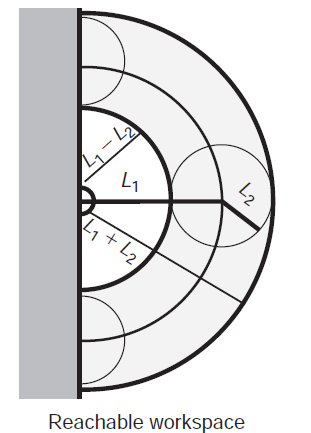L2的末端的坐标位置确定之后，L1和L2臂对应的两个关节偏转角度和就可以通过几何方法确定了。

令L2的末端的最终坐标位置为(X,Y)，连接首关节和尾关节后，可以分析出最终解应该有两个，这两个解关于直线(0,0)到(X,Y)对称。

最终可以使用余弦定理来计算出最终的和，推导过程如下：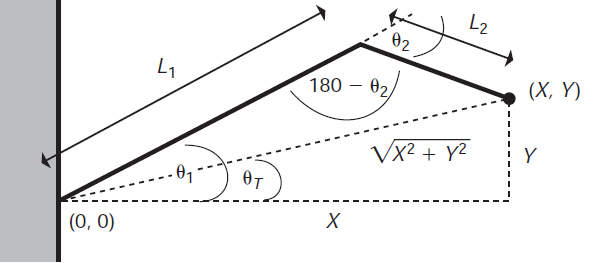因此有：

余弦定理：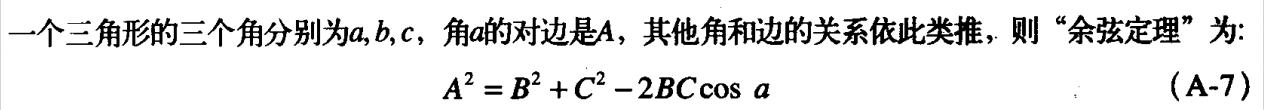余弦定理：

根据余弦定理，将值代入公式得

根据余弦定理：

有：

这样得到了关节旋转角度和，注意的是cos函数是偶函数，因此需要考虑到±θ的情况。

运动学正解（Forward kinematics）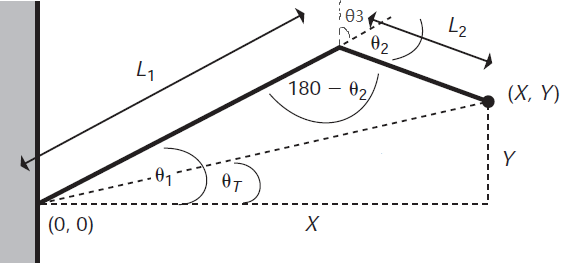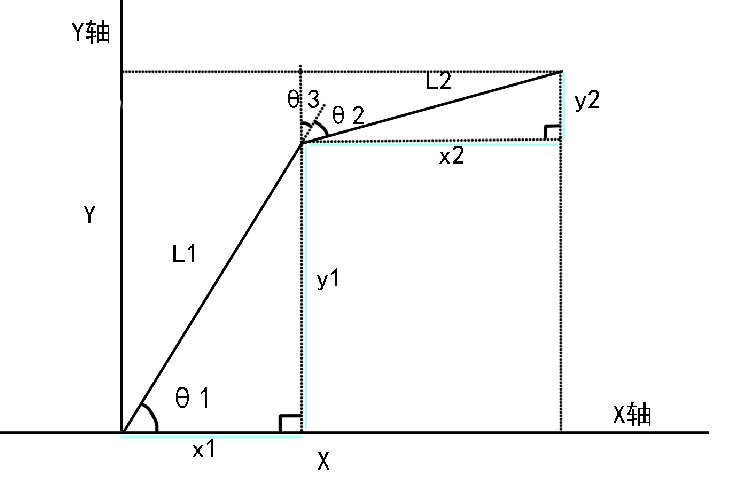x1,x2,y1,y2分别是蓝色线段部分

由于x1和x2是平行的，所以L1和x1所成角度，和L1和x2所成角度相同，所以有

根据三角函数:

则

代入上式得

代入上式得

软件模拟实现

根据上面的推导的几何公式，写一个模拟的2d机械臂试试看,软件用的processing。

PVector P = new PVector(20,50);
//Version 1.1-fix coordinate

//坐标轴偏移坐标
float coordsetoffx,coordsetoffy;

//IK运算，大小臂夹角，平面投影直线夹角
float [] IKrst  = new float;

//大小臂长度
float bigL = 200,smallL = 200;

class arm
{
//单臂建模为射线
//位置，方向，长度，坐标偏移
PVector position;
PVector vector;
float L;
float coordsetoffx,coordsetoffy;

arm(PVector a,float lengths)
{
vector = new PVector(random(-1,1),random(-1,1)).normalize();
position = a;
L = lengths;
coordsetoffx = 0;
coordsetoffy = 0;
}

arm(PVector a,float lengths,float cx,float cy)
{
vector = new PVector(random(-1,1),random(-1,1)).normalize();
position = a;
L = lengths;
coordsetoffx = cx;
coordsetoffy = cy;

}

//设置方向向量
void setVector(PVector a)
{
vector = a.normalize();
}

//设置位置
void setPosition(PVector a)
{
position = a;
}

//获取臂末端位置
PVector getLPosition()
{

return PVector.add(position,PVector.mult(vector,L));

}
//绘制臂
void display()
{
PVector temp = PVector.mult(vector,L);
line(coordsetoffx+position.x,coordsetoffy+position.y,coordsetoffx+position.x+temp.x,coordsetoffy+position.y+temp.y);

}

}

//向量坐标变换,将y值翻转
PVector tranPvector(PVector a)
{
//return new PVector(a.x,-a.y,a.z);
return new PVector(a.x,-a.y);
}

//IK
float [] IK(float x,float y,float L1,float L2)
{
//依次是平面直线夹角，大臂夹角，小臂夹角
float ctaT;
float cta1;
float cta2;

float [] ctasuzu  = new float;

ctaT = acos(x/sqrt(x*x+y*y));
//如果坐标0以下翻转角度加减
if(y < 0)
{
ctaT = -ctaT;
}

cta1 = acos((L1*L1+x*x+y*y-L2*L2)/(2*L1*sqrt(x*x+y*y)))+ctaT;
cta2 = acos((x*x+y*y-L1*L1-L2*L2)/(2*L1*L2));

ctasuzu = ctaT;
ctasuzu = cta1;
ctasuzu = cta2;

return ctasuzu;

}

//声明arm类的2个变量
arm arm1,arm2,arm3;

void setup()
{
size(700,700);
//坐标偏移取窗口中心
coordsetoffx = width/2;
coordsetoffy = height/2;

//大臂位置，长度，坐标偏移，
arm1 = new arm(new PVector(0,0),bigL,coordsetoffx,coordsetoffy);
//方向
arm1.setVector(tranPvector(new PVector(0.5,1)));

//将大臂的末端位置给小臂，臂长，坐标偏移
arm2 = new arm(arm1.getLPosition(),smallL,coordsetoffx,coordsetoffy);

//knift head
arm3 = new arm(arm2.getLPosition(),20,coordsetoffx,coordsetoffy);

background(255);
}

void draw()
{
background(255);

//映射鼠标到坐标系
IKrst = IK(map(mouseX,0,width,-width/2,width/2),map(mouseY,height,0,-height/2,height/2),bigL,smallL);

println("position: "+map(mouseX,0,width,-width/2,width/2)+" "+map(mouseY,height,0,-height/2,height/2));
println("C: "+degrees(IKrst)+" "+degrees(IKrst)+" "+degrees(IKrst)+" ");
//println("arm2:"+arm2.getLPosition().x,arm2.getLPosition().y);
//println("arm1:"+arm1.getLPosition().x,arm1.getLPosition().y);

float xx = cos(IKrst);
float yy = sin(IKrst);

float xx2 = cos((IKrst-IKrst));
float yy2 = sin((IKrst-IKrst));

arm1.setVector(tranPvector(new PVector(xx,yy)));

arm2.setPosition(arm1.getLPosition());
arm2.setVector(tranPvector(new PVector(xx2,yy2)));

arm3.setPosition(arm2.getLPosition());
arm3.setVector(tranPvector(new PVector(xx2,yy2)));

stroke(0);
arm1.display();
arm2.display();
stroke(255,0,0);
arm3.display();

noStroke();
fill(0,128,0);
ellipse(coordsetoffx+arm2.getLPosition().x,coordsetoffy+arm2.getLPosition().y,10,10);

fill(255,0,0);
ellipse(coordsetoffx+arm3.getLPosition().x,coordsetoffy+arm3.getLPosition().y,10,10);

}

void mousePressed()
{

}

实现效果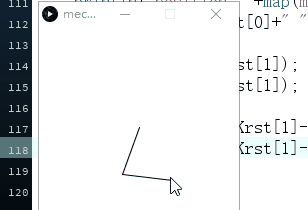2维的机械臂运动学原理知道了，接下来讲讲3维的3轴机械臂的运动学正反解。

第二篇:grbl控制3轴机械臂 原理 实现 （二） 之3D机械臂模拟及实现


展开全文GRBL
• 通过前面的知识了解后，接下来实现GRBL的源码修改，让其支持机械臂。 我们先找到GRBL的源码下载下来 下载地址：github源码地址 我用的是grbl0.9版本，主要文件有这些： （robot_arm.c这个文件是我们新增的） ...
往期回顾：

第一篇:grbl控制3轴机械臂 原理 实现 （一） 之2D机械臂模拟及实现

第二篇:grbl控制3轴机械臂 原理 实现 （二） 之3D机械臂模拟及实现

第三篇:grbl控制3轴机械臂 原理 实现 （三） 之如何通过步进电机控制机械臂、插补算法

通过前面的知识了解后，接下来实现GRBL的源码修改，让其支持机械臂。

我们先找到GRBL的源码下载下来    下载地址：github源码地址

我用的是grbl0.9版本，主要文件有这些：

（robot_arm.c这个文件是我们新增的）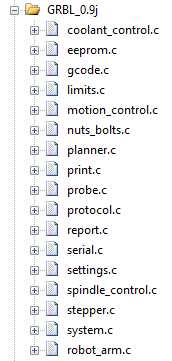接线来看看，要改这里面哪些文件

先打开setting.c文件，由于机械臂需要初始化，我们通过GRBL的$命令来传初始化参数，所以新增几个命令 找到settings_store_global_setting函数 在 case 27: settings.homing_pulloff = value; break;后面加上 //ROBOT_ARM //$100=0 like x = 0;change current position value
case 30:
sys.position[X_AXIS] = lroundf(value*settings.steps_per_mm[X_AXIS]);
plan_sync_position();//同步平台总步长,传入大臂角度，算出总步长
return(STATUS_OK);	 //直接返回 不储存到flash

case 31:
sys.position[Y_AXIS] = lroundf(value*settings.steps_per_mm[Y_AXIS]);
plan_sync_position();//同步平台总步长,传入小臂角度，算出总步长
return(STATUS_OK);	//直接返回 不储存到flash

case 32:
sys.position[Z_AXIS] = lroundf(value*settings.steps_per_mm[Z_AXIS]);
plan_sync_position();//同步平台总步长,传入旋转角度，算出总步长
return(STATUS_OK);	//直接返回 不储存到flash

case 33:
SCARA_LINKAGE_1 = value; //传入机械臂大臂臂长
return(STATUS_OK);  //直接返回 不储存到flash

case 34:
SCARA_LINKAGE_2 = value; //传入机械臂小臂臂长
return(STATUS_OK);  //直接返回 不储存到flash

打开config.h 定义一下机械臂的宏定义，随意地方加上

//定义robotarm
#define ROBOT_ARM 1

打开system.c文件，找到system_convert_array_steps_to_mpos函数将里面的内容替换成

  uint8_t idx;

#if defined (IS_ROBOT_ARM)
float position_scara;
for (idx=0; idx<N_AXIS; idx++)
{
//角度 = 脉冲/多少脉冲每1度
position_scara[idx] = system_convert_axis_steps_to_mpos(steps, idx);
}
//角度通过前向运动，运算出坐标
//fixed small big arm no belong
forward_kinematics_ROBOT(position_scara,position);

#else
for (idx=0; idx<N_AXIS; idx++) {
position[idx] = system_convert_axis_steps_to_mpos(steps, idx);
}
#endif
return;

打开limits.c文件，找到函数limits_go_home 在里面的    } while (n_cycle-- > 0);前面加上

#if defined (ROBOT_ARM)
scara_report_positions();
#endif

在其 } while (n_cycle-- > 0); 后面更改为

  for (idx=0; idx<N_AXIS; idx++) {
// NOTE: settings.max_travel[] is stored as a negative value.
if (cycle_mask & bit(idx)) {
#if defined (IS_ROBOT_ARM)
set_axis_position = lroundf((ManualHomePos[idx]+settings.homing_pulloff)*settings.steps_per_mm[idx]);
angle_mode = false;
#elif defined (HOMING_FORCE_SET_ORIGIN)
set_axis_position = 0;
#else
if ( bit_istrue(settings.homing_dir_mask,bit(idx)) ) {
set_axis_position = ((settings.max_travel[idx]+settings.homing_pulloff)*settings.steps_per_mm[idx])>0?(int32_t)((settings.max_travel[idx]+settings.homing_pulloff)*settings.steps_per_mm[idx]+0.5):(int32_t)((settings.max_travel[idx]+settings.homing_pulloff)*settings.steps_per_mm[idx]-0.5);
} else {
set_axis_position = (-settings.homing_pulloff*settings.steps_per_mm[idx])>0?(int32_t)(-settings.homing_pulloff*settings.steps_per_mm[idx]+0.5):(int32_t)(-settings.homing_pulloff*settings.steps_per_mm[idx]-0.5);
}
#endif

打开planner.c,找到函数 plan_buffer_line 为其添加变量

  int32_t target_steps[N_AXIS];
float unit_vec[N_AXIS], delta_mm;
uint8_t idx;
float inverse_unit_vec_value;
float inverse_millimeters;  // Inverse millimeters to remove multiple float divides
float junction_cos_theta;
float sin_theta_d2;

#if defined (IS_ROBOT_ARM)
float target_float[N_AXIS];
int32_t position_steps[N_AXIS];
#endif

  // Prepare and initialize new block
plan_block_t *block = &block_buffer[block_buffer_head];
block->step_event_count = 0;
block->millimeters = 0;
block->direction_bits = 0;
block->acceleration = SOME_LARGE_VALUE; // Scaled down to maximum acceleration later
#ifdef USE_LINE_NUMBERS
block->line_number = line_number;
#endif

// Copy position data based on type of motion being planned.
//根据计划的运动类型复制位置数据。
#if defined (IS_ROBOT_ARM)
memcpy(position_steps, pl.position, sizeof(pl.position));
#endif

其下方继续添加

#if defined (IS_ROBOT_ARM)
//通过逆运动学，将目标坐标target换算成机械臂三轴的角度target_float
//fixed small big arm no belong
inverse_kinematics_ROBOT(target,target_float);
#endif


其下面的for循环改成

  for (idx=0; idx<N_AXIS; idx++) {
// Calculate target position in absolute steps, number of steps for each axis, and determine max step events.
// Also, compute individual axes distance for move and prep unit vector calculations.
// NOTE: Computes true distance from converted step values.
#if defined (COREXY)
if ( !(idx == A_MOTOR) && !(idx == B_MOTOR) ) {
target_steps[idx] = (target[idx]*settings.steps_per_mm[idx])>0?(int32_t)(target[idx]*settings.steps_per_mm[idx]+0.5):(int32_t)(target[idx]*settings.steps_per_mm[idx]-0.5);
block->steps[idx] = labs(target_steps[idx]-pl.position[idx]);
}
block->step_event_count = max(block->step_event_count, block->steps[idx]);
if (idx == A_MOTOR) {
delta_mm = ((target_steps[X_AXIS]-pl.position[X_AXIS]) + (target_steps[Y_AXIS]-pl.position[Y_AXIS]))/settings.steps_per_mm[idx];
} else if (idx == B_MOTOR) {
delta_mm = ((target_steps[X_AXIS]-pl.position[X_AXIS]) - (target_steps[Y_AXIS]-pl.position[Y_AXIS]))/settings.steps_per_mm[idx];
} else {
delta_mm = (target_steps[idx] - pl.position[idx])/settings.steps_per_mm[idx];
}

#elif defined (IS_ROBOT_ARM)
//target是轴移动的距离，单位是毫米，系统设定了steps_per_mm值，也就是每毫米代表的轴移动步数，直接换算
//得到轴移动步数target_steps
//公式： 此轴总共要走的脉冲数 =  角度 * 多少脉冲/1度
target_steps[idx] = lroundf(target_float[idx]*settings.steps_per_mm[idx]);
//target表示轴从原点移动到终点的总距离，所以当前线段的移动步数需要用target减去之前所有线段移动的总步数
//得到相对移动的脉冲数
block->steps[idx] = abs(target_steps[idx]-position_steps[idx]);
//获得三个轴里移动距离最远的轴移动的距离，后面DDA直线插补时会用到这个值。关于DDA插补算法的方法后面会介绍
block->step_event_count = max(block->step_event_count, block->steps[idx]);
//根据步数换算出真实移动的距离，保存在unit_vec中，后面计算两条线段夹角时会用到
//公式： 移动的相对角度 = （轴移动总脉冲 - 之前移动脉冲的和）即相对脉冲数 / 多少脉冲/1度
delta_mm = (target_steps[idx] - position_steps[idx])/settings.steps_per_mm[idx];

#else
target_steps[idx] = (target[idx]*settings.steps_per_mm[idx])>0?(int32_t)(target[idx]*settings.steps_per_mm[idx]+0.5):(int32_t)(target[idx]*settings.steps_per_mm[idx]-0.5);
block->steps[idx] = labs(target_steps[idx]-pl.position[idx]);
block->step_event_count = max(block->step_event_count, block->steps[idx]);
delta_mm = (target_steps[idx] - pl.position[idx])/settings.steps_per_mm[idx];

#endif
unit_vec[idx] = delta_mm; // Store unit vector numerator. Denominator computed later.

打开gcode.c文件，gc_execute_line函数里面，在case 61下面添加

          case 61:
word_bit = MODAL_GROUP_G13;
if (mantissa != 0) { FAIL(STATUS_GCODE_UNSUPPORTED_COMMAND); } // [G61.1 not supported]
// gc_block.modal.control = CONTROL_MODE_EXACT_PATH; // G61
break;

#if defined (IS_ROBOT_ARM)
case 95:
angle_mode=!angle_mode;
break;
#endif

新建robot_arm.h 和 robot_arm.c文件

robot_arm.h

#ifndef ROBOT_ARM_h
#define ROBOT_ARM_h

#include "grbl.h"

#ifdef ROBOT_ARM
#define IS_ROBOT_ARM 1
#endif

// Length of inner and outer support arms. Measure arm lengths precisely.
//内外支撑臂的长度。精确测量臂长。

//#define SCARA_LINKAGE_1 200.0f //mm   大臂
//#define SCARA_LINKAGE_2 200.0f //mm   小臂 最外侧末端

// SCARA tower offset (position of Tower relative to bed zero position)
// This needs to be reasonably accurate as it defines the printbed position in the SCARA space.
//SCARA塔偏移（塔相对于床零位的位置）
//这需要相当准确，因为它定义了SCARA空间中打印床的位置。

//robotarm
#define SCARA_OFFSET_X 0 //mm 200
#define SCARA_OFFSET_Y 0 //mm
#define SCARA_OFFSET_Z 0 //mm

#define MANUAL_X_HOME_POS -45.43f
#define MANUAL_Y_HOME_POS 95.67f
#define MANUAL_Z_HOME_POS 0.0f

#define RADIANS(d) ((d)*(float)M_PI/180.0f)
#define DEGREES(r) ((r)*180.0f/(float)M_PI)

#define sq(x) x*x

extern volatile float SCARA_LINKAGE_1; //mm   大臂
extern volatile float SCARA_LINKAGE_2; //mm   小臂 最外侧末端

extern volatile float X_error_correction; //输入的长度/实际走长度
extern volatile float Y_error_correction; //
extern volatile float Z_error_correction; //

extern bool angle_mode;
extern float ManualHomePos;

void scara_report_positions(void) ;

//fixed small big arm no belong
void inverse_kinematics_ROBOT(float const *cartesian, float *f_scara);
void forward_kinematics_ROBOT(float const *f_scara, float *cartesian);

#endif


robot_arm.c

#include "grbl.h"
#include "robot_arm.h"

volatile float SCARA_LINKAGE_1 = 200.0f; //mm   大臂
volatile float SCARA_LINKAGE_2 = 200.0f; //mm   小臂 最外侧末端

volatile float X_error_correction = 1; 		//输入的长度/实际走长度
volatile float Y_error_correction = 1;
volatile float Z_error_correction = 1;

#define L1  SCARA_LINKAGE_1
#define L2  SCARA_LINKAGE_2
#define L1_2  sq(SCARA_LINKAGE_1)
#define L2_2  sq(SCARA_LINKAGE_2)

//float const L1 = SCARA_LINKAGE_1,
//            L2 = SCARA_LINKAGE_2,
//            L1_2 = sq(SCARA_LINKAGE_1),
//            L2_2 = sq(SCARA_LINKAGE_2);

bool angle_mode=false;

float ManualHomePos={MANUAL_X_HOME_POS,MANUAL_Y_HOME_POS,MANUAL_Z_HOME_POS};

//此正向运动时根据大臂,小臂都是独立角度，即大臂移动后，小臂角度对应平面不变
//传入值 f_scara是大臂和小臂的角度，cartesian是最终求得的坐标(这里输入的小臂角度是，平面垂线到小臂的角度)
//输入角度 返回坐标
void forward_kinematics_ROBOT(float const *f_scara, float *cartesian)
{
/***********************robot arm****************************/
float X,Y,Z;
float project3D;
//f_scara里面的0,1,2代表：大臂角度，小臂角度，旋转角度

//         z +  /y
//           | /
//           |/
//           +-----+x

//2d机械臂朝向的方向
//Y = cos(RADIANS(f_scara[X_AXIS])) * L1 + sin(RADIANS(f_scara[Y_AXIS]) + RADIANS(90) - RADIANS(f_scara[X_AXIS])) * L2;

//3D 两臂投影到平台平面长度,通过三角函数转化为坐标轴方向长度
//cos(a) * L1 + sin(b) * L2
project3D = cos(RADIANS(f_scara[X_AXIS])) * L1 + sin(RADIANS(f_scara[Y_AXIS])) * L2;
Y = cos(RADIANS(f_scara[Z_AXIS])) * project3D;

//垂直向上的方向
//sin(a) * L1 + cos(b) * L2
Z = sin(RADIANS(f_scara[X_AXIS])) * L1 + cos(RADIANS(f_scara[Y_AXIS])) * L2;

//2d站机械臂后方看向它，它的右边方向
//X = sin(RADIANS(f_scara[Z_AXIS])) * cos(RADIANS(f_scara[X_AXIS])) * L1 + sin(RADIANS(f_scara[Y_AXIS]) + RADIANS(90) - RADIANS(f_scara[X_AXIS])) * L2;

//3D 站机械臂后方看向它，它的右边方向
X = sin(RADIANS(f_scara[Z_AXIS])) * project3D;

cartesian[X_AXIS] = X + SCARA_OFFSET_X;  //求得用户坐标系下X值
cartesian[Y_AXIS] = Y + SCARA_OFFSET_Y;  //求得用户坐标系下Y值
cartesian[Z_AXIS] = Z + SCARA_OFFSET_Z;

/***********************robot arm****************************/

}

//此正向运动时根据大臂,小臂都是独立角度，即大臂移动后，小臂角度对应平面不变
// f_scara是大臂和小臂的角度,和整体旋转角度，cartesian是坐标(这里的小臂角度是，平面垂线到小臂的角度)
//输入坐标 返回角度
void inverse_kinematics_ROBOT(float const *cartesian, float *f_scara)
{
/***********************robot arm****************************/

//         z +  /y
//           | /
//           |/
//           +-----+x

static float ROBOTARM_alpha, ROBOTARM_beta, ROBOTARM_cta ,ROBOTARM_alphapsi, projectxyLength, X, Y, X_2,Y_2,sqrtx_2ay_2;

//实际测试机械臂的世界坐标下Y轴有误差，输入长度/实际要走长度  比值 0.76
//如果不是角度模式
if(!angle_mode)
{
//实际要走路程
f_scara[X_AXIS] = f_scara[X_AXIS] / X_error_correction;
f_scara[Y_AXIS] = f_scara[Y_AXIS] / Y_error_correction;
f_scara[Z_AXIS] = f_scara[Z_AXIS] / Z_error_correction;
}

//首先求得目标点 到 原点的距离
//获取机械臂投射到xy平面的长度
//length = sqrt(x*x + y*y)
projectxyLength = sqrtf(sq(cartesian[X_AXIS]) + sq(cartesian[Y_AXIS]));
//将3d机械臂变量变为2d机械臂的变量
//	projectxyLength长度为2d机械臂的X
X = projectxyLength;
X_2 = sq(X);
Y = cartesian[Z_AXIS];
Y_2 = sq(Y);
sqrtx_2ay_2 = sqrtf(X_2 + Y_2);
//求得机械臂所在yz旋转平面的alphapsi角度
ROBOTARM_alphapsi = acosf(X / sqrtx_2ay_2);
//如果坐标在平面以下，将alphapsi取反
if (Y < 0)
{
ROBOTARM_alphapsi = -ROBOTARM_alphapsi;
}
//求得机械臂所在yz旋转平面的alpha角度,，即大臂到xy平面的角度(实际是弧度)
ROBOTARM_alpha = acosf((L1_2 + X_2 + Y_2 - L2_2) / (2 * L1 * sqrtx_2ay_2) ) + ROBOTARM_alphapsi;
//求得小臂的角度(实际是弧度)
ROBOTARM_beta = acosf((X_2 + Y_2 - L1_2 - L2_2) / (2 * L1 * L2) );
//由于小臂是相对大臂不动的，换算成小臂相对平面垂线的角度
ROBOTARM_beta = RADIANS(90) - ROBOTARM_alpha + ROBOTARM_beta;

//求得整体机械臂的旋转角度(实际是弧度)
ROBOTARM_cta = atan2f(cartesian[X_AXIS] , cartesian[Y_AXIS]);

//如果不是角度模式
if(!angle_mode)
{
f_scara[X_AXIS] = DEGREES(ROBOTARM_alpha); //大臂旋转弧度转换为角度
f_scara[Y_AXIS] = DEGREES(ROBOTARM_beta);   //小臂旋转弧度转换为角度
f_scara[Z_AXIS] = DEGREES(ROBOTARM_cta);

//test robot_arm
printPgmString("\r\n IK degrees  ");
for (int idx=0; idx< N_AXIS; idx++) {
printFloat_CoordValue(f_scara[idx]);
if (idx < (N_AXIS-1)) { printPgmString(","); }
}

}
//否则是角度模式
else
{
f_scara[X_AXIS] = cartesian[X_AXIS];
f_scara[Y_AXIS] = cartesian[Y_AXIS];
f_scara[Z_AXIS] = cartesian[Z_AXIS];

}

/***********************robot arm****************************/

}

void scara_report_positions()
{
u8 idx;
static float position_scara;

for (idx=0; idx<N_AXIS; idx++)
{
position_scara[idx] = system_convert_axis_steps_to_mpos(sys.position, idx);
}
printPgmString("ROBOTARM ALPHA:");
printFloat(position_scara[X_AXIS],2);
printPgmString("   ROBOTARM Beta:");
printFloat(position_scara[Y_AXIS],2);
printPgmString("   ROBOTARM CTA:");
printFloat(position_scara[Z_AXIS],2);
printPgmString("\n");

}



打开grbl.h添加robot_arm.h头文件

#if defined (ROBOT_ARM)
#include "robot_arm.h"
#endif

至此文件修改完毕，接下来编译，烧录到芯片中就行了。步进电机接线时注意电机方向，反了对调A+ A-线就行了。

接下来我们试试用机械臂来写个字，这里用到软件有inkscape和grbl controler，这里提供这两款打包好的下载链接：软件

首先我们生成要写的字的G代码，打开inkscape软件。

点击文件->文档属性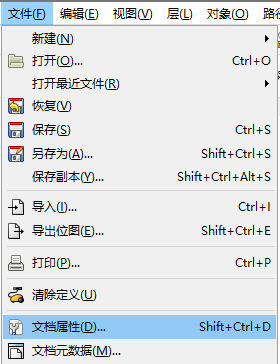我们将单位改成mm，长宽都设为100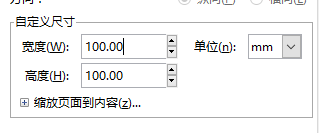点击左侧的文字编辑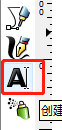然后在画布上打上你要的字体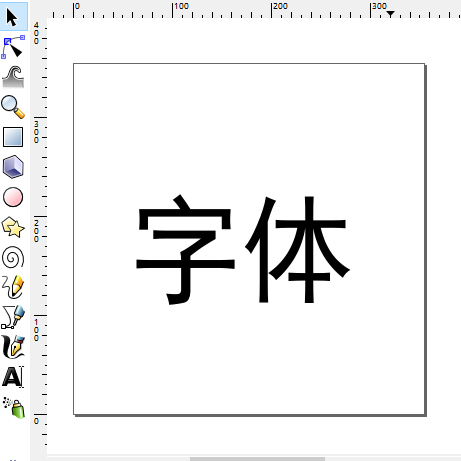点击这个字体选中它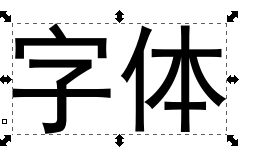点击菜单的  路径->对象转化成路径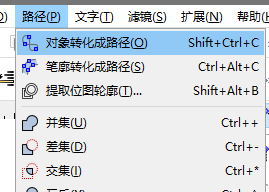然后，点击菜单的  扩展->Laserengraver->Laser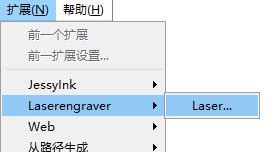点击perferences，设置好输出文件路径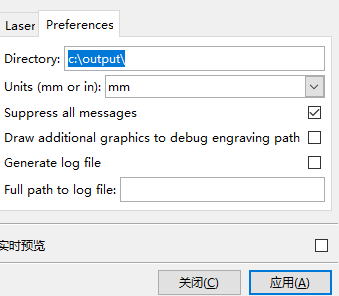到Laser调好速度，输出文件名字，点击应用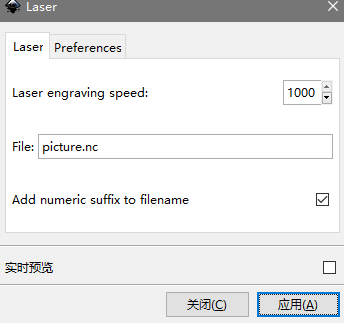能够看到生成了字体笔画轨迹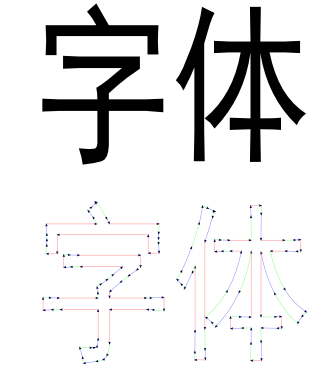这样就会得到一个文件picture.nc,我们用记事本打开它

我们把所有的M05字符都换成 G1  Z5，即z方向向上移动5mm，作为抬笔动作

将M03 替换成 G1  Z0 ，作为落笔动作。

保存退出

把我们的机械臂，大臂小臂摆好后再上好电，最好这样摆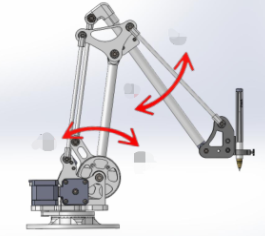我们可以用手机的水平仪量出a和b的角度，量出大臂和小臂的长度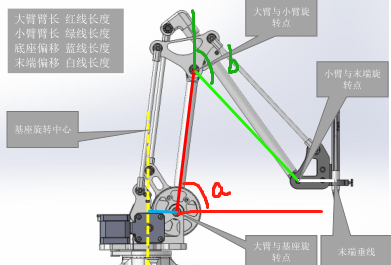我们打开GRBLcontroller软件，连接上串口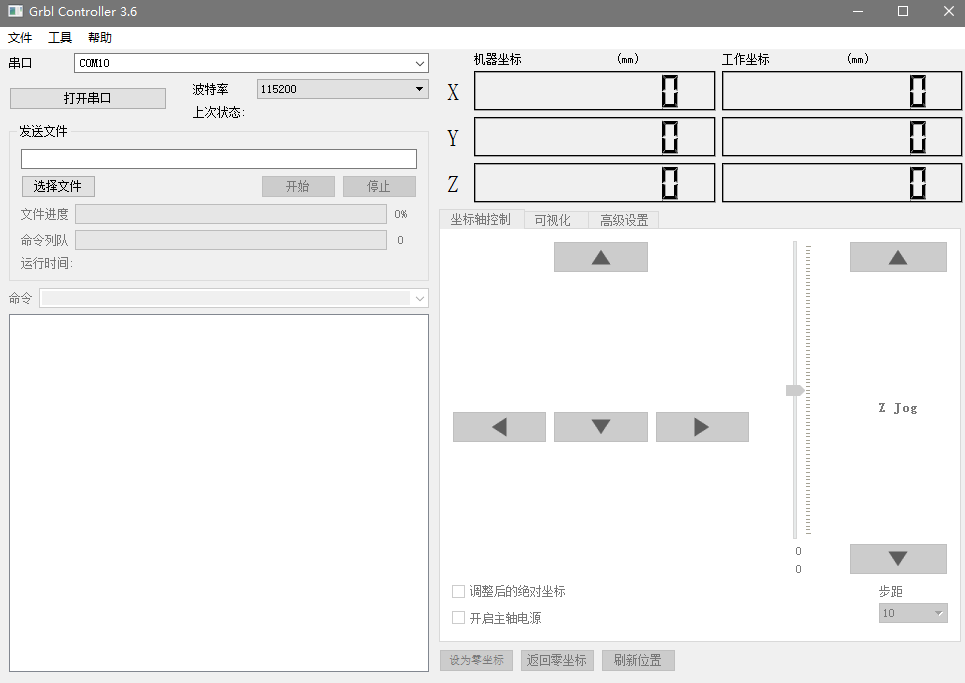连上后发初始化指令，校准机械臂的位置。这里的值根据你自己测量相应填入

$30=85 即大臂a角度$31=130           即小臂b角度

$32=0 即旋转角度$33=200           即大臂长度

\$34=200           即小臂长度

然后选好步距，通过这几个箭头，慢慢调整机械臂的笔头到要写字的平面附近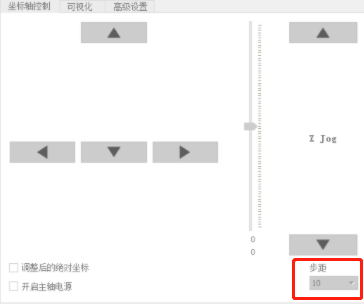调整好后，点击设为零坐标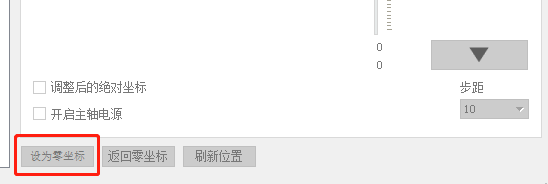现在就可以开始写字了，点击选择文件，选刚刚的picture.nc文件，点击开始，机械臂就开始动起来了。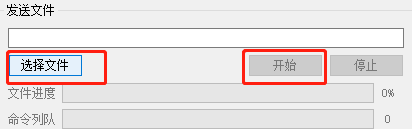写出来的字体效果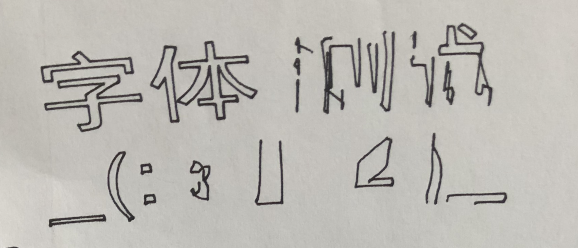我们可以看到，左边字体效果良好，而右边写的不好，这是因为机械臂的结构原因，导致臂之间连接是有个松动范围的，用弹簧把各个机械臂往任意一侧拉扯住，就不会造成臂的滑动了，当时我是用手直接掰着它把它给固定住的。

前期测试，其他效果不好的图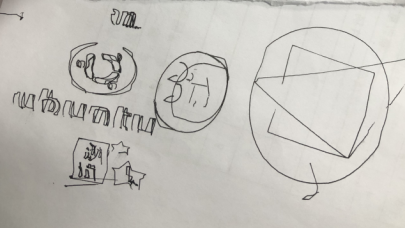展开全文GRBL
• 前面我们用运动学逆解求出了机械臂的三个角度，那么我们让机械臂对应的电机转动所求得的角度就能得到机械臂的最终姿态了。由于是转动固定角度，要最终姿态保持和预料的一样的话，那么机械臂的初始位置就很重要，要...
参考：

图形学入门

https://www.zhihu.com/question/20330720

https://zhuanlan.zhihu.com/p/30553006

https://www.cnblogs.com/soroman/archive/2006/07/27/509602.html

https://blog.csdn.net/tlvc/article/details/4913397

https://blog.csdn.net/u010141928/article/details/79514514

往期回顾：

第一篇:grbl控制3轴机械臂 原理 实现 （一） 之2D机械臂模拟及实现

第二篇:grbl控制3轴机械臂 原理 实现 （二） 之3D机械臂模拟及实现

步进电机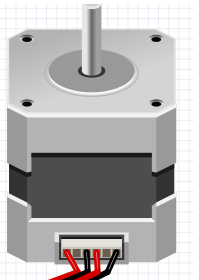这里机械臂上所用的步进电机是常用的42步进电机，每个电机控制一个关节，步进角度1.8度，全步模式下，200个脉冲走360度。这里我们在步进电机上面加了减速器，减速比是1:10，即转子真正走一圈是要2000个脉冲，每个脉冲是走0.18度。

前面我们用运动学逆解求出了机械臂的三个角度，那么我们让机械臂对应的电机转动所求得的角度就能得到机械臂的最终姿态了。由于是转动固定角度，要最终姿态保持和预料的一样的话，那么机械臂的初始位置就很重要，要确保机械臂的初始位置已知与准确。比如，大臂的初始位置处于30度，最终姿态是要跑到60度的位置，即步进电机要增加30度，如果初始位置不是处于30度的位置，那么增加30度后最终位置就不会是60度。所以我们在机械臂上电初始化时，就要有个变量保存三个角度的值，以及一个初始位置的校准，确保实际机械臂的角度和变量中的角度值是对应与准确的。

知道上面的知识后，那么要控制机械臂就简单了。说白了就是控制X,Y,Z这3个电机走相应的角度。这里与原版的笛卡尔坐标系控制电机就有点不一样了，在笛卡尔坐标系下，每个电机都对应一个轴，要走到对应的坐标点，分别给对应轴对应的值就行了。比如坐标系的目标点在(0,0,10),那么只要Z轴电机移动10就行了。

而机械臂要走空间坐标系的话就不是这种对应关系，更具逆解的值，要对应的操作3个电机移动不同的角度。所以我们这里要做一些相应的转换。将距离、角度、步数（步进电机要走的步数，即脉冲数）对应起来。

公式： 此轴总共要走的脉冲数 =  角度 * 多少脉冲每1度

这里角度我们已经知道了，而多少脉冲每1度（根据电机驱动设置的细分值，和对应电机的步进角度，以及减速器决定）举例：如果电机的细分为全步，就是1细分，步进角度为1.8度，减速器为1:10。

那么  一个脉冲所走度数 = 细分*步进角度*减速比

多少脉冲每1度  =  (360度 / (细分*步进角度*减速比)) / 360度

代入即得   得出值是5.5555555555....

由此得出每个轴要走的脉冲数，控制电机走对应的脉冲就行了。

关于所走的距离，可以通过运动学正解求出起始点与终点的位置来得到。

如果我们要让机械臂走出直线，即在空间坐标系中任意两点以直线轨迹移动，这里就要涉及到插补了。

插补

由于我们用的是步进电机，运动的时候是一步一步的，并不是平滑的，这里假设在一个平面上面，一个x步进电机，一个y步进电机。那么放大它们所走的轨迹就会是阶梯状的。而它们也只能沿着网格运动。相当于有了一个最小的分辨率，这个分辨率就是一步。如下图所示，如果x，y电机同时走就能走出45度的斜线。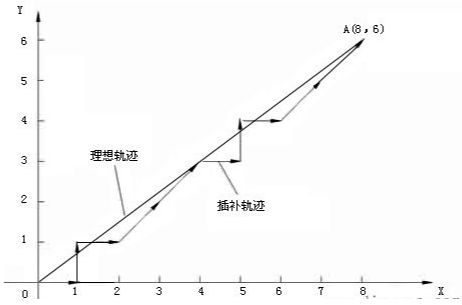这里所用的算法和图形学有点关系，我们的显示屏都是像素点，那么如何在这些像素网格上画出直线呢？

图形学的画线算法如何运用到，步进电机上呢？我们可以把一像素看成是步进的一步，这样像素每移动一格，对应步进电机就移动一步。

下面一一介绍

直线插补

数值微分DDA(Digital Differential Analyzer)算法

直线方程：

递增坐标x来求出y的值，假设知道了

那么为：

联合上面的得

也就是说每次纵坐标都是在前一个纵坐标的基础上加上斜率k的值得到的，这样运算中少了乘法，运算速度就加快了。

这里用processing编程演示一下

void settings()
{
//设置显示屏幕窗口大小
size(150,150);
}

//DDA画线算法
void DDADrawLine(int x0,int y0,int x1,int y1)
{
//算出斜率k值
float k = (y1-y0)*1.0/(x1-x0);
//起始点为y0
float y = y0;

//循环范围x0-x1，每次递增k值
for(int x = x0 ; x <= x1 ; ++ x) {
//画下一个像素点，y值四舍五入
setpixelsCT(x,(int)round(y));
//y值递增k
y += k;
}
}

//由于processing的屏幕坐标是左上角为原点，x向右递增，y轴向下递增
//为了适配数学上的坐标轴，做了y轴调转
void setpixelsCT(int x,int y)
{
loadPixels();
pixels[((height-1)-y)*xx+x] = color(0);
updatePixels();
}

void draw()
{
//背景显示白色
background(255);

//鼠标映射，为了适配数学上的坐标轴，做了y轴调转
DDADrawLine(0,0,mouseX,(int)map(mouseY,height-1,0,0,height-1));

}

程序演示：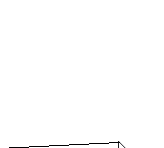Bresenham算法

在效率上，虽然DDA消除了浮点的乘法运算，但是存在浮点加法和取整运算。1965年Bresenham提出了更好的直线生成算法，成为了时至今日图形学领域使用最广泛的直线生成算法。该算法采用增量计算，借助一个误差量的符号确定下一个像素点的位置，该算法中不存在浮点数，只有整数运算，大大提高了运行效率。

Bresenham的思想是将像素中心构造成虚拟网格线，下图中每个红点即是一个像素。计算所画线条与垂直网格线的交点，算出与之相隔最近的像素坐标。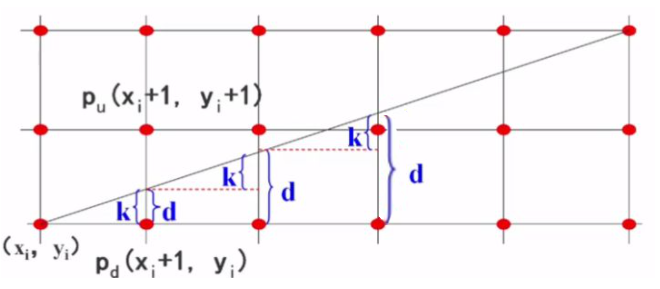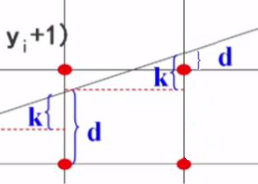和DDA算法一样，每次x加1的时候纵方向就加了k，保存一个d值，如果d小于等于0.5，那么就画在下面的点，如果d大于0.5就画在上面的点。每次检查d是否在[0,1)范围内，如果不在此范围d减1。d值减一和y的像素坐标会上移一个单位，此时再次从这个新位置计算d值(如上面第二幅图)。

按这思想，无优化代码如下

void BresenhamDrawLine(int x0,int y0,int x1,int y1)
{

//根据直线方程算出k值
float k = (y1-y0)*1.0/(x1-x0);

//初始化y值坐标值
int y = y0;

//初始化d值
float d = 0;

//循环绘制像素点 从x0到x1
for(int x = x0 ; x <= x1 ; ++ x)
{
//如果d值大于0.5像素，表明上面的像素离直线比较近，采用直线上面的点，否则采用下面的点
if(d > 0.5)
{
//绘制一个像素点,采用直线上面的点,将y值加1
setpixelsCT(x,y+1);
}
else
{
//绘制一个像素点
setpixelsCT(x,y);
}

//如果d值大于1个像素，将标志归零重置，d减一,y值加一像素
if(d >= 1.0)
{
d -= 1.0;
y++;
}

//当x每移动一像素，累加k值到d
d += k;

}

}



接下来优化下，去掉除法和小数运算

消除除法和小数,把d值偏移到0就只需要判断正负号，两边同时乘以2和dx（乘以2是为了去掉0.5小数，乘以dx是为了k值消除除法）

我们已知直线斜率是

而d的值是  是由，由k值累加得到的，d值最终要与0.5来比较，得出是大于还是小于0.5，由此得出取直线上面的像素还是下面的像素。

所以我们先去掉除法，这里直线的起点,和终点都是整数。

我们直接在k值上乘以一个,消除k值的除数 得到

又由于要消掉0.5这个小数，所以要乘以2。

那么k值现在为

与d值比较的0.5  和 1.0在乘以后得到     和

那么最终代码为：

void LiteBresenhamDrawLine(int x0,int y0,int x1,int y1)
{

//根据直线方程算出k值
int dx = abs(x0-x1);
int dy = abs(y0-y1);

//初始化y值坐标值
int y = y0;

//消除除法和小数,由于只需要判断正负号，两边同时乘以2和dx（乘以2是为了去掉0.5小数，乘以dx是为了k值消除除法）所以得出
//k = dy/dx;
//2*dx*k = 2*dx*dy/dx
int k = 2*dy;

//初始化d值,由于d值是k的累加，这里也要乘以2dx
//d = 0
//d = d - 0.5
//这样初始值就是-0.5，一旦d累加增k值大于0，等同于d>0.5,只是整体偏移了-0.5，这样就能看符号判断是否大于或小于0.5了
//接下来去掉小数，乘以2dx，得到初始值,实际偏移了dx
int d = -dx;

//循环绘制像素点 从x0到x1
for(int x = x0 ; x <= x1 ; ++ x)
{
//如果d值大于0.5像素，表明上面的像素离直线比较近，采用直线上面的点，否则采用下面的点
//由于都乘以了2dx，所以这里和它比较的0.5也要乘以2dx，成了2*dx*0.5=dx，实际偏移了dx所以为0
if(d > 0)
{
//绘制一个像素点,采用直线上面的点,将y值加1
setpixelsCT(x,y+1);
}
else
{
//绘制一个像素点
setpixelsCT(x,y);
}

//如果d值大于1个像素，将标志归零重置，d减一,y值加一像素
//由于都乘以了2dx，所以这里和它比较的1.0也要乘以2dx，成了2*dx*1.0=2dx，实际偏移了dx所以为dx
if(d >= dx)
{
d -= 2*dx;
y++;
}

//当x每移动一像素，累加k值到d
d += k;

}

}

以上示例代码都是基于k值范围在(0,1],要适用所有象限的话，只要在第一象限求出坐标后对应改变x，y值的符号后再绘制即可实现。

圆弧插补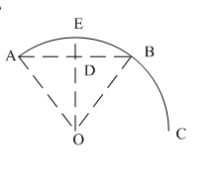grbl中的圆弧插补是采用直线逼近法，即采用弦高（ED）很小的弦线（直线AB）替代圆弧（圆弧AB）。

（R半径，ED弦高，已知值）

认为直线AB的长度等于圆弧AB的弧长，由弧长公式：弧长=弧长对应的圆心角*半径

可以计算出圆心角（OA与OB的夹角），A点坐标为已知，所以可以计算出B点坐标，然后使用直线插补AB线段，使用AB线段代替AB弧。

圆插补

中点画圆法，考虑圆心在原点，半径为R的圆在第一象限内的八分之一圆弧，从点（0, R）到点（R/ , R/ ）顺时针方向确定这段圆弧。假定某点Pi(xi, yi)已经是该圆弧上最接近实际圆弧的点，那么Pi的下一个点只可能是正右方的P1或右下方的P2两者之一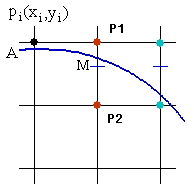构造判别函数：

F(x, y）= x2 + y2 – R2

当F(x, y）= 0，表示点在圆上，当F(x, y）> 0，表示点在圆外，当F(x, y）< 0，表示点在圆内。如果M是P1和P2的中点，则M的坐标是（xi + 1, yi – 0.5），当F（xi + 1, yi – 0.5）< 0时，M点在圆内，说明P1点离实际圆弧更近，应该取P1作为圆的下一个点。同理分析，当F（xi + 1, yi – 0.5）> 0时，P2离实际圆弧更近，应取P2作为下一个点。当F（xi + 1, yi – 0.5）= 0时，P1和P2都可以作为圆的下一个点，算法约定取P2作为下一个点。

现在将M点坐标（xi + 1, yi – 0.5）带入判别函数F(x, y），得到判别式d：

d = F（xi + 1, yi – 0.5）= (xi + 1)2 + (yi – 0.5)2 – R2

若d < 0，则取P1为下一个点，此时P1的下一个点的判别式为：

d’ = F（xi + 2, yi – 0.5）= (xi + 2)2 + (yi – 0.5)2 – R2

展开后将d带入可得到判别式的递推关系：

d’ = d + 2xi + 3

若d > 0，则取P2为下一个点，此时P2的下一个点的判别式为：

d’ = F（xi + 2, yi – 1.5）= (xi + 2)2 + (yi – 1.5)2 – R2

展开后将d带入可得到判别式的递推关系：

d’ = d + 2(xi - yi) + 5

特别的，在第一个象限的第一个点（0, R）时，可以推倒出判别式d的初始值d0：

d0 = F(1, R – 0.5) = 1 – (R – 0.5)2 – R2 = 1.25 - R

根据上面的分析，可以写出中点画圆法的算法。考虑到圆心不在原点的情况，需要对计算出来的坐标进行了平移，下面就是通用的中点画圆法的processing源代码：

int xx,yy;

void settings()
{
xx=250;
yy=250;
size(xx,yy);
}

void MP_Circle(int xc , int yc , int r)
{
int x, y;
double d;
x = 0;
y = r;
d = 1.25 - r;
CirclePlot(xc , yc , x , y);
while(x < y)
{
if(d < 0)
{
d = d + 2 * x + 3;
}
else
{
d = d + 2 * ( x - y ) + 5;
y--;
}
x++;
CirclePlot(xc , yc , x , y);
}
}

//coordnate transform
void setpixelsCT(int x,int y)
{
loadPixels();
pixels[((height-1)-y)*xx+x] = color(0);
updatePixels();
}

//整个圆绘制
void CirclePlot(int X,int Y,int P,int Q)
{
setpixelsCT(X + P, Y + Q);
setpixelsCT(X - P, Y + Q);
setpixelsCT(X + P, Y - Q);
setpixelsCT(X - P, Y - Q);
setpixelsCT(X + Q, Y + P);
setpixelsCT(X - Q, Y + P);
setpixelsCT(X + Q, Y - P);
setpixelsCT(X - Q, Y - P);
}

void draw()
{
background(255);

//画圆
MP_Circle(125,125,50);
}

改进的中点画圆法－Bresenham算法

中点画圆法中，计算判别式d使用了浮点运算，影响了圆的生成效率。如果能将判别式规约到整数运算，则可以简化计算，提高效率。于是人们针对中点画圆法进行了多种改进，其中一种方式是将d的初始值由1.25 – R改成1 – R，考虑到圆的半径R总是大于2，因此这个修改不会响d的初始值的符号，同时可以避免浮点运算。还有一种方法是将d的计算放大两倍，同时将初始值改成3 – 2R，这样避免了浮点运算，乘二运算也可以用移位快速代替，采用3 – 2R为初始值的改进算法，又称为Bresenham算法：

int xx,yy;

void settings()
{
xx=250;
yy=250;
size(xx,yy);
}

void Bresenham_Circle(int xc , int yc , int r)
{
int x, y, d;

x = 0;
y = r;

d = 3 - 2 * r;

CirclePlot(xc , yc , x , y);
while(x < y)
{
if(d < 0)
{
d = d + 4 * x + 6;
}
else
{
d = d + 4 * ( x - y ) + 10;
y--;
}
x++;
CirclePlot(xc , yc , x , y);
}

}

//coordnate transform
void setpixelsCT(int x,int y)
{
loadPixels();
pixels[((height-1)-y)*xx+x] = color(0);
updatePixels();
}

//整个圆绘制
void CirclePlot(int X,int Y,int P,int Q)
{
setpixelsCT(X + P, Y + Q);
setpixelsCT(X - P, Y + Q);
setpixelsCT(X + P, Y - Q);
setpixelsCT(X - P, Y - Q);
setpixelsCT(X + Q, Y + P);
setpixelsCT(X - Q, Y + P);
setpixelsCT(X + Q, Y - P);
setpixelsCT(X - Q, Y - P);
}

void draw()
{
background(255);

//画圆
Bresenham_Circle(125,125,50);
}

绘制效果：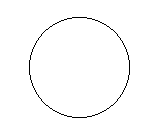通过以上的基础知识了解，终于可以进入正题了，下面讲讲GRBL源码如何修改得能控制机械臂

第四篇:grbl控制3轴机械臂 原理 实现 （四） 之GRBL源码修改驱动三轴机械臂


展开全文GRBL
• % 三连杆机械臂瞬态运动的牛顿-欧拉递归逆动力学求解： % 参数：（运动指令）各关节运动角度， 关节速度， 关节加速度（3*1矩阵） % 返回值：各关节力矩（3*1矩阵） function tau = min_Three_link_manipulator...matlab 数学建模
• 6轴机械臂 3D打印资料 3D打印图纸 6轴机械臂——AR2.zip
• 轴机械臂调试入门一、安装接线1.机箱2.控制柜接线二、机械臂调试1.打开机械臂制动器2.打开ui调试工具3.插电开机 一、安装接线 1.机箱 机箱如图所示： 主机箱背后连接电源线，网线（连接电脑主机）和act/lnn接口...ubuntu
• 硬件平台 机器人手臂使用ABB的120型号的六轴机械手臂 使用d652板卡与三菱fx3g plc 进行点位数据交互，由plc控制器对机械手臂进行控制。
• 对于3轴码垛机械臂控制最基本的是对其建立运动学模型，而对于3轴码垛类型机械臂来说运动学模型，其本质就是给定空间3D坐标，求解3个轴的旋转角度。 如上图所示，左侧为实物坐标，右侧图为抽象到坐标系的几何表示，...树莓派
• 6轴机械臂正逆解运算实现 利用Gluon-6L3机械臂模型的参数（参数来源于知乎@梁政），对机械臂进行运动学分析。 这里采用标准DH坐标系，并将d6设置为0，方便后续计算。 首先，SDH的变换矩阵为： ii−1T=Ai=^{i-1}_iT=A...
• 继上次写博客已经过去一周了，我终于把机械臂的机械本体建完模了，不多说，先上图 ...机械臂的头部包括第五和第六，采用9g舵机作为动力，整体框架由3mm的两块侧板和两块支撑板组成，支撑板与管状材料通过502粘..经验分享 c++ qt
• 使用材料： ...4轴机械臂 舵机 LM2596 降压模块 电池 #include <Servo.h> // 声明调用Servo.h库 Servo myservo1;// 创建一个舵机对象 Servo myservo2; Servo myservo3; Servo myservo4...
• 本文件是基于LabVIEW2013，其中包含有4自由度机械臂仿真几种问题：关节反解 三次插值 五次插值 用曲线表现出各个关节的位置，角度，角速度，同时给出三维空间内五次插值 的运动轨迹 其中的3D图像是采用子VI的方式...
• 【从零开始的ROS四轴机械臂控制】（一）- 实际模型制作、Solidworks文件转urdf与rviz仿真 一、模型制作 1.实际模型制作 2.Solidworks模型制作 二、Solidworks文件转urdf 1.sw_urdf_exporter插件 2.添加坐标系和转轴 ...ros python vmware arduino
• robot_->place(place_, place_, place_, place_, place_, place_, vel_, acc_, vscale_, appr_); #endif place_pose_ = nullptr; } rclcpp::shutdown(); return 0; } place_publisher....
• 轴机械臂从下位机（arduino）+上位机（ros+moveit） 目录 一、机械臂的硬件 1、机械部分 2、电控部分 二、机械臂的下位机搭建 1、控制器接线 2、注意事项 三、机械臂的上位机搭建 1、ROS环境的搭建 2...ROS gazebo
• 我是一个目录基于MATLAB的关节型六轴机械臂轨迹规划仿真1 实验目的2 实验内容2.1标准D-H参数法2.2实验中使用的Matlab函数3实验结果4 全部代码 基于MATLAB的关节型六轴机械臂轨迹规划仿真 1 实验目的 基于机器人学...数学建模 matlab
• | 3| q3| 0| 200| -1.5708| 0| | 4| q4| 640| 0| 1.5708| 0| | 5| q5| 228| 0| -1.5708| 0| | 6| q6| 0| 0| 0| 0| +---+-----------+-----------+-----------+-----------+-----------+ 2、MDH建模仿真 ： L1...
• python IDE编辑器Sublime Text 3 (3211) 简介 Sublime Text 是一个文本编辑器（收费软件，可以无限期试用，但是会有激活提示弹窗），同时也是一个先进的代码编辑器。Sublime Text是由程序员Jon Skinner于2008年1月份...windows
• 今天，我将向您介绍MPU-6050（加速度计+陀螺仪）传感器模块，以及如何通过它控制由微型伺服电机制成的简单2轴机械臂。 InvenSense MPU-6050是一款低成本，高精度，六自由度（DOF）的惯性测量单元（IMU ）。IMU可以...
• do3 y2 di3 x3 do4 y3 di4 x4 do5 y4 di5 x5 do6 y5 di6 x6 do7 y6 di7 y7 di8 plc输入 输入描述 plc输出 输出描述 x10 夹取气缸到位 y10 夹取气缸 x11 放料气缸到位 y11 放料气缸 x12 ...
• 6轴机械臂3个主轴（基本轴）用以保证末端执行器达到工作空间的任意位置，3个次轴（腕部轴）用以返回实现末端执行器的任意空间姿态。 2 坐标系 大部分商用工业机器人系统中，均可使用关节坐标系、直角坐标系、...
• 【从零开始的ROS四轴机械臂控制（四）】七、图像处理节点1.节点功能与实现方法2.iamge_process 相关程序部分程序解释3.节点运行与测试 七、图像处理节点 1.节点功能与实现方法 我们的仿真环境已经搭建好了，接下来...python opencv 计算机视觉 经验分享
• 制作时长：3天制作成本：<200元难度系数：容易过去的一年小伙伴在QQ群内提了不少问题，其中最多应该是如何制作机械臂了。《利用舵机制作简单机械臂》这篇文章已经发布了几年，它是基于S...
• 创建机械臂模型 一、准备工作 1）创建工作空间,在src下创建arm功能包 2）建立文件夹launch和urdf分别用来存放.launch文件和.xacro文件 ...因此，虚拟一个六轴机械臂。 编写机械臂的URDF文件，为后续M.python
• ## 【机械臂】机械臂快速入门

万次阅读 多人点赞 2018-04-02 14:18:53
六自由度解释 除了空间内的三个坐标数据（确定位置xyz），还需要确定所选点的姿态，所以需要六个自由度才能达到空间的任意位置，例如五自由度，能绕三个转动但只能沿着xy平移，5自由度可以指定姿态但只能沿着xy...
• ur3机械臂sw转urdf文件 1.安装sw_urdf_exporter插件并加载ur3模型 sw_urdf_exporter插件网址:http://wiki.ros.org/sw_urdf_exporter 安装时关闭sw,一路默认安装就可以了. 2.插入基准 插入六根基准，六根也是此...linux
• Faze4是可完全3d打印的小型开源6轴机械臂。 它在功能和美学上都与工业机械手臂类似，但主要用于研究，教育和任何有兴趣制造自己的机械手臂的人。 该手臂与其他DIY手臂分开的主要“卖点”是： <1000美元便宜。 ...C++
• 因此，我用lego的EV3砖块和伺服器构建了一个6轴机械臂。 现在我需要软件来控制它。 要将手臂的尖端移动到所需位置，我只想在手臂可触及范围内的三维空间中指定该点。 人工智能应该为我完成所有艰苦的工作。 开始 我...JavaScript...